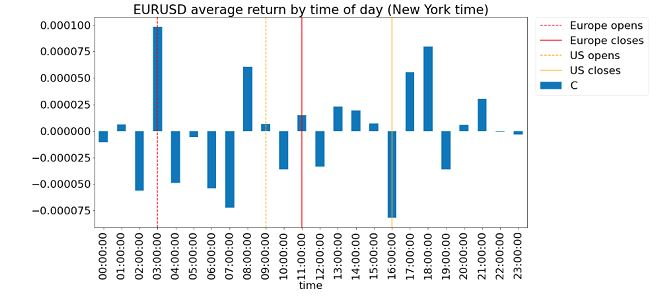## Minggu, 01 Februari 2009

### Kelly formula revisited

Some discussions on Kelly's formula with a reader Steven L:

Q:
"I am more than half way through your book and am stuck at a concept that I can't seem to find an answer in any other forum.

I have read Ralph Vince's "Portfolio Management Formulas," which uses Kelly's formula to calculate an optimal "fraction" of the bankroll to bet on each trial. So a trader can calculate a fraction of his total trading account value to risk on each trade. What I am referring to is the so-called "fixed-fractional" trading. There exists an optimal fraction that will maximize the geometric growth rate of the trading equity, in theory anyway.

However, in the money management chapter of your book, you use Kelly's formula to derive an optimal "leverage." This seems to be in conflict with what I learned from Ralph Vince, since leverage is usually great than unity and fraction is usually less than unity. I can't seem to make a connection between these two concepts. I have also seen the same optimal leverage formula in Lars Kestner's Quantitative Trading Strategies and asked the same question on some forums, but no one was able to give me a clear satisfactory answer. It would be greatly helpful if you can help me sort out the confusion."
A:

I don't have Ralph Vince's book with me, but if I recall correctly, his formulation is based on discrete bets (win or lose, no intermediate outcome), much like horse-betting or in a casino game. My approach, or rather, Professor Ed Thorp's approach, is based on continuous finance, assuming that every second, your P&L could fluctuatate in a Gaussian ("log-normal") fashion.

For discrete bets where you could have lost all of your equity in one bet, surely one should only bet a fraction of your total equity. For continuous finance, there is very little chance one could have lost all of the equity in one time period, due to the assumed log-normal distribution of prices. Hence one should bet more than your equity, i.e. use leverage.

Q:

In example 6.2 in your book, the portfolio consists of only long SPY, which has little chance of going to zero. So I can see how it is reasonable that you use the continuous finance approach and apply the optimal leverage to scale up the return.

But let's assume that the portfolio consists of a single strategy that buys options. Suppose this strategy will lose most of the time due to time decay but will make profit once in a while due to black-swan events. I don't think it's a good idea to bet the entire portfolio equity on each trade for this strategy. Can you still apply the continuous finance approach in this case, since in reality trading is like making discreet bets? Should we expect the mean and variance of this strategy automatically result in an Optimal Leverage that is less than one? So that we actually need to risk a fraction of the account equity per trade?
A:

The formula I depicted in the book is valid only if the P&L distributions are Gaussian. If one expects a fat-tailed distribution due to black-swan events, a different mathematical model needs to be used, though it can still be within the continuous finance framework. However, for simplicity's sake, if the distribution looks multinomial (e.g. high probability of "Win a lot" v "Lose a lot"), then you may model it with fractional betting just like a casino game.